Question

A transverse, wave travelling on a chord is represented by D=0.22sin (5.6x+34t) where D and x are inmeters and t is in seconds. For this wave, determine; a) wavelength b) frequency c) velocity (both magnitude and direction) d) amplitude e) maximum and minimum speed of particles in the chord.

1.a) λ = 1.12 m

b) f = 5.41 Hz

c) v = 154.54 m/s

d) A = 0.22m

e)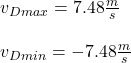Explanation:

You have the following equation for a wave traveling on a cord: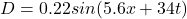(1)

The general expression for a wave is given by: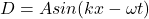(2)

By comparing the equation (1) and (2) you have:

A: amplitude of the wave = 0.22m

k: wave number = 5.6 m^-1

w: angular velocity = 34 rad/s

a) The wavelength is given by substitution in the following expression: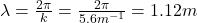b) The frequency is: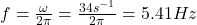c) The velocity of the wave is: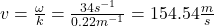d) The amplitude is 0.22m

e) To calculate the maximum and minimum speed of the particles you obtain the derivative of  the equation of the wave, in time: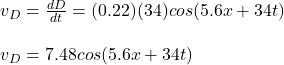cos function has a minimum value -1 and maximum +1. Then, you obtain for maximum and minimum velocity: